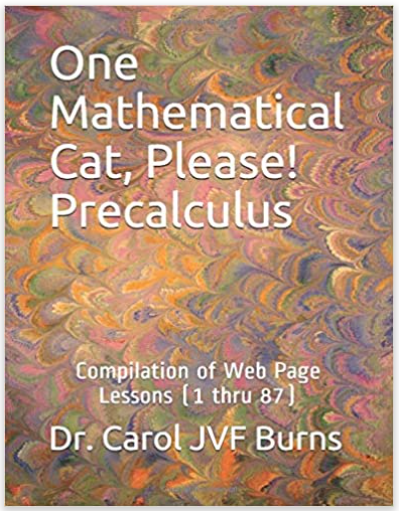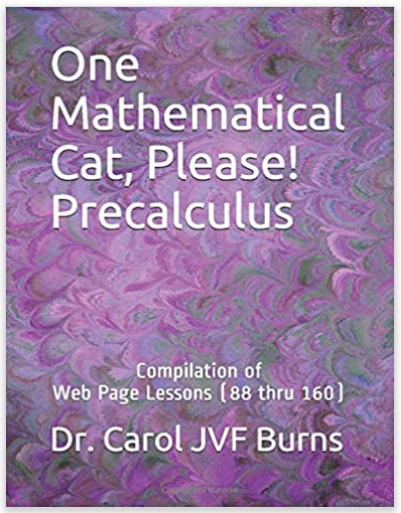# One Mathematical Cat, Please! PRECALCULUS

This is a complete, sequenced, free course.

It has unlimited, randomly-generated exercises
and worksheets in every lesson.

It can be used for a year-long high school course
or a one-semester college course.
Or, just enjoy it yourself, at your own pace!lessons 1–87lessons 88–160
get a print version
of these lessons

 1 prerequisites: language of mathematics essentials 2 prerequisites: function review; difference quotients 3 solving linear inequalities in one variable 4 solving nonlinear inequalities in one variable (introduction) 5 the test point method for sentences like ‘$f(x) \gt 0$’ 6 the test point method for sentences like ‘$f(x) \gt g(x)$’ 7 the test point method ‘in a nutshell’ and additional practice 8 absolute value as distance from zero 9 absolute value as distance between two numbers 10 circles and the ‘completing the square’ technique 11 review of lines and slopes of lines 12 distance between points; the midpoint formula 13 sketching regions in the coordinate plane 14 testing equations for symmetry 15 more on difference quotients 16 finding the domain and range of a function 17 working with linear functions: finding a new point, given a point and a slope 18 increasing and decreasing functions 19 reading information from the graph of a function 20 basic function models you must know 21 piecewise-defined functions 22 direct and inverse variation 23 proportionality problems 24 What is the graph of $\,y = f(x)\,$? 25 shifting graphs up/down/left/right 26 horizontal and vertical stretching/shrinking 27 reflecting about axes, and the absolute value transformation 28 multi-step practice with all the graphical transformations 29 even and odd functions 30 extreme values of functions (max/min) 31 review of quadratic functions: vertex form, max/min, intercepts, more 32 max/min problems resulting in quadratic functions 33 combining functions to get new functions 34 composition of functions 35 writing a function as a composition 36 using a function box ‘backwards’ 37 one-to-one functions 38 ‘undoing’ a one-to-one function; inverse functions 39 properties of inverse functions 40 finding inverse functions (when there's only one $x$ in the formula) 41 finding inverse functions (switch input/output names method) 42 the graph of an inverse function 43 introduction to polynomials 44 relationship between the zeros (roots) and factors of polynomials 45 end behavior of polynomials 46 turning points of polynomials 47 long division of polynomials 48 the division algorithm 49 synthetic division 50 the remainder theorem 51 ‘trapping’ the roots of a polynomial; an interval guaranteed to contain all real roots 52 introduction to complex numbers 53 arithmetic with complex numbers 54 the square root of a negative number 55 the complex conjugate 56 the quadratic formula revisited; the discriminant 57 multiplicity of zeros and graphical consequences 58 discussion: graphing polynomials with technology (optional; no exercises) 59 Solve an equation? Find a zero? Your choice! 60 the fundamental theorem of algebra 61 polynomials with real number coefficients 62 solving polynomial equations 63 introduction to rational functions 64 introduction to asymptotes 65 introduction to puncture points (holes) 66 finding vertical asymptotes 67 finding ‘puncture points’ of graphs 68 finding horizontal asymptotes 69 finding slant asymptotes 70 exponential functions: review and additional properties 71 linear versus exponential functions 72 recognizing linear and exponential behavior from tables of data 73 the natural exponential function 74 introduction to average rate of change 75 introduction to instantaneous rate of change and tangent lines 76 a special property of the natural exponential function 77 (optional) a surprising appearance of the irrational number  e ; diluting a toxic liquid 78 simple versus compound interest 79 continuous compounding 80 logarithmic functions: review and additional properties 81 logarithm summary: properties, formulas, laws 82 exponential growth and decay: introduction 83 exponential growth and decay: relative growth rate 84 solving exponential equations 85 solving logarithmic equations 86 solving exponential growth and decay problems 87 doubling time, half-life 88 introduction to trigonometry 89 the right triangle approach to trigonometry 90 the unit circle approach to trigonometry 91 compatibility of the right triangle and unit circle approaches 92 special triangles and common trigonometric values 93 reference angles 94 radian measure: associating real numbers with points on the unit circle 95 the trigonometric functions 96 signs of all the trigonometric functions 97 trigonometric values of special angles 98 fundamental trigonometric identities 99 periodic functions 100 the period of a periodic function 101 graphs of sine and cosine 102 graphing generalized sine and cosines, like $y = a\sin k(x\pm b)$ and $y = a\cos (kx\pm B)$ 103 amplitude, period, and phase shift 104 given amplitude, period, and phase shift, write an equation 105 graph of the tangent function 106 graph of the secant function 107 review of circles (and related concepts) 108 length of a circular arc (and related concepts) 109 (optional) the angle subtended by a geometric object at an external point 110 area of a circular sector 111 sine and cosine as scaling factors of hypotenuse 112 area of a triangle 113 law of sines 114 Given two sides and a non-included angle, how many triangles? 115 law of cosines 116 (optional) revisiting ‘equal’ versus ‘approximately equal’ 117 using the Law of Cosines in the SSS case (& introduction to the arccosine function) 118 summary: solving triangles (all types) 119 verifying trigonometric identities 120 addition and subtraction formulas for sine and cosine 121 double-angle formulas for sine and cosine 122 trying to ‘undo’ trigonometric functions 123 inverse trigonometric function: arcsine 124 inverse trigonometric function: arccosine 125 inverse trigonometric function: arctangent 126 inverse trigonometric function problems: all mixed up 127 solving simple trigonometric equations 128 solving pseudo-quadratic equations 129 introduction to vectors 130 working with the arrow representation for vectors 131 working with the analytic representation for vectors 132 unit vectors 133 formula for the length of a vector 134 vectors: from direction/magnitude to horizontal/vertical components 135 vectors: from horizontal/vertical components to direction/magnitude 136 vector application: finding true speed and direction 137 vector application: forces acting an an object in equilibrium 138 introduction to partial fraction expansion/decomposition (PFE) 139 PFE: linear factors 140 PFE: irreducible quadratic factors 141 introduction to conic sections 142 identifying conics by the discriminant 143 (optional) intersecting an infinite double cone and a plane: looking at the equations 144 (optional) How is it that the conic discriminant tells us the type of conic? 145 parabolas: definition, reflectors/collectors, derivation of equations 146 finding the equation of a parabola 147 definition of an ellipse 148 reflecting property of an ellipse 149 equations of ellipses in standard form: foci on the $\,x$-axis 150 (optional) getting an ellipse by stretching/shrinking a circle 151 (optional) two useful transforms: reflection about the line $\,y = x\,$ and counterclockwise rotation by $\,90^\circ$ 152 equations of ellipses in standard form: foci on the $\,y$-axis 153 summary: equations of ellipses in standard form 154 definition of a hyperbola 155 equations of hyperbolas in standard form 156 graphing hyperbolas 157 reflecting property of a hyperbola 158 shifting conics (shifting any equation in two variables) 159 polar coordinates 160 parametric equations